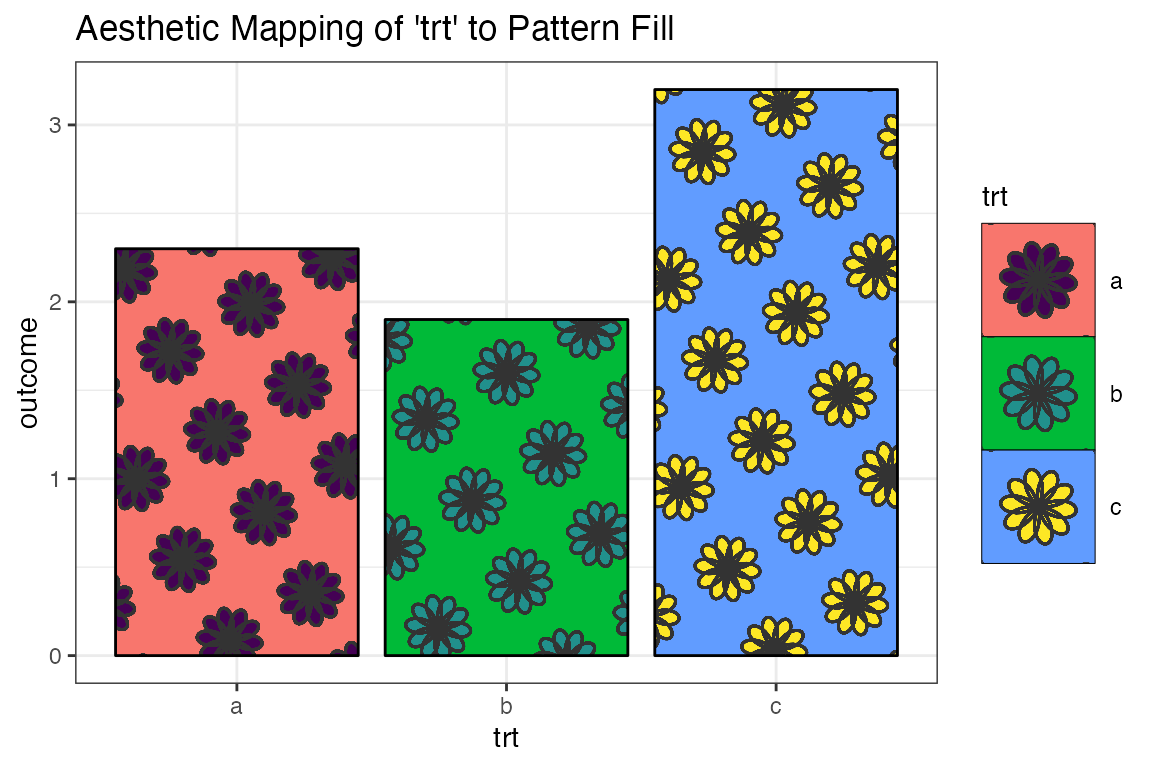suppressPackageStartupMessages({
library(ggplot2)
library(ggpattern)
})

## Introduction to the ‘circle’, ‘pch’, ‘regular_polygon’, and ‘rose’ patterns

• The ‘circle’ pattern draws circles

• The ‘pch’ pattern draws “plotting character” symbols as in graphics::points()

• The ‘regular_polygon’ pattern draws convex/star regular polygons

• The ‘rose’ pattern draws rose curves

## Pattern Parameters

aesthetic description default possible values
pattern_colour Stroke colour ‘grey20’ colour
pattern_fill Fill colour ‘grey80’ colour
pattern_angle Rotation angle 30 angle in degrees
pattern_density Approx. fraction of area the pattern fills 0.2 value in range [0, 1] (fraction)
pattern_spacing Spacing between repetitions of pattern 0.05 value in range [0, 1] (snpc units)
pattern_xoffset Shift pattern along x axis 0 value in range [0, 1] (snpc units)
pattern_yoffset Shift pattern along y axis 0 value in range [0, 1] (snpc units)
pattern_alpha Alpha NA value in range [0, 1] or NA
pattern_linetype Stroke linetype 1 linetype
pattern_size Stroke linewidth 1 linewidth
pattern_type Pattern type NA See circle, pch, regular_polygon, and rose pattern documentation
pattern_subtype Pattern subtype NA See circle, pch, regular_polygon, and rose pattern documentation
pattern_grid Grid type ‘square’ ‘square’, ‘hex’, or ‘hex_circle’
pattern_rot Pattern symbol rotation angle 0 angle in degrees
pattern_shape Pattern shape NA See pch and regular_polygon
pattern_frequency Frequency 0.1 Angular frequency in ‘rose’ pattern

There are also a number of parameters for extra control of legend sizing and aspect ratio adjustments. See the ‘Pattern Parameters - Common’ for more information.

## Data

Standard data for all the example plots

df <- data.frame(trt = c("a", "b", "c"), outcome = c(2.3, 1.9, 3.2))
df
#>   trt outcome
#> 1   a     2.3
#> 2   b     1.9
#> 3   c     3.2

## Vanilla ggplot2

ggplot(df, aes(trt, outcome)) +
geom_col(aes(fill=trt),colour='black') +
theme_bw() +
labs(title = "Plain ggplot2")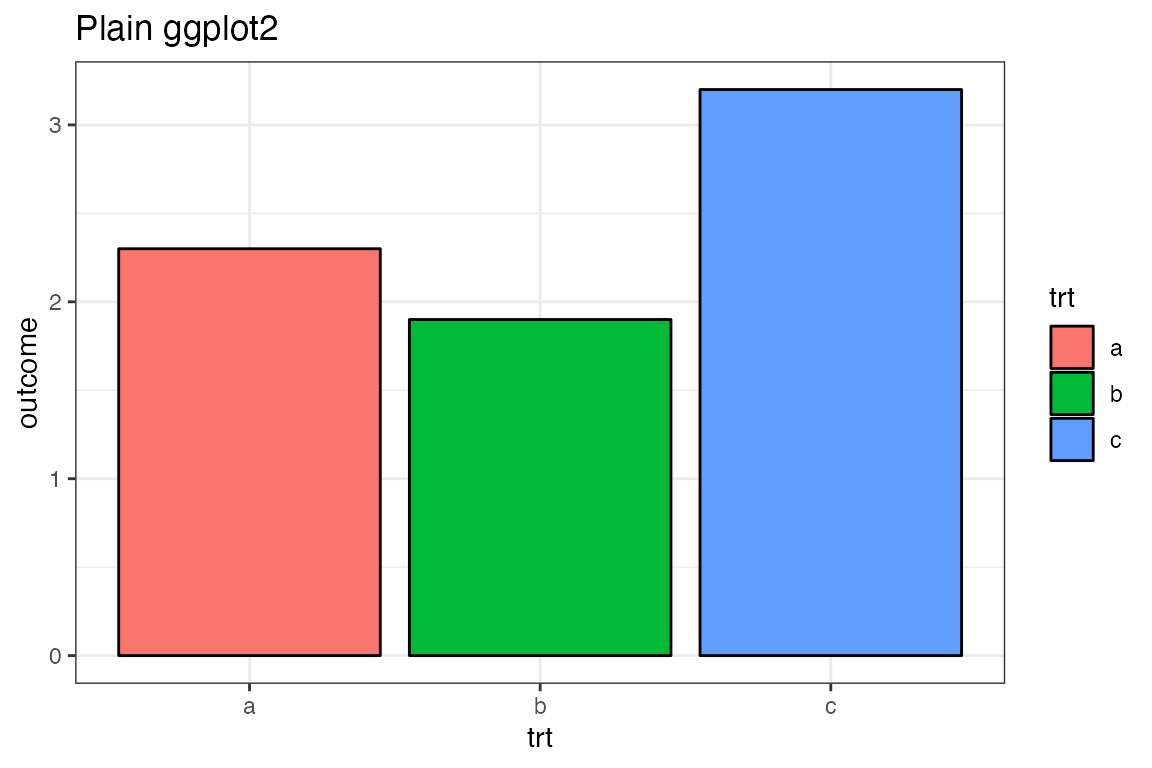## Use the {ggpattern} geom

• Use ggpattern::geom_col_pattern() instead of ggplot2::geom_col().
• Set pattern = 'circle'
• Default legends in ggplot2 are usually too small to show off an example of the pattern, so it will usually be necessary to increase the key size.
ggplot(df, aes(trt, outcome)) +
geom_col_pattern(aes(fill=trt),colour='black', pattern = 'circle') +
theme_bw() +
labs(title = "ggpattern") +
theme(legend.key.size = unit(1.5, 'cm'))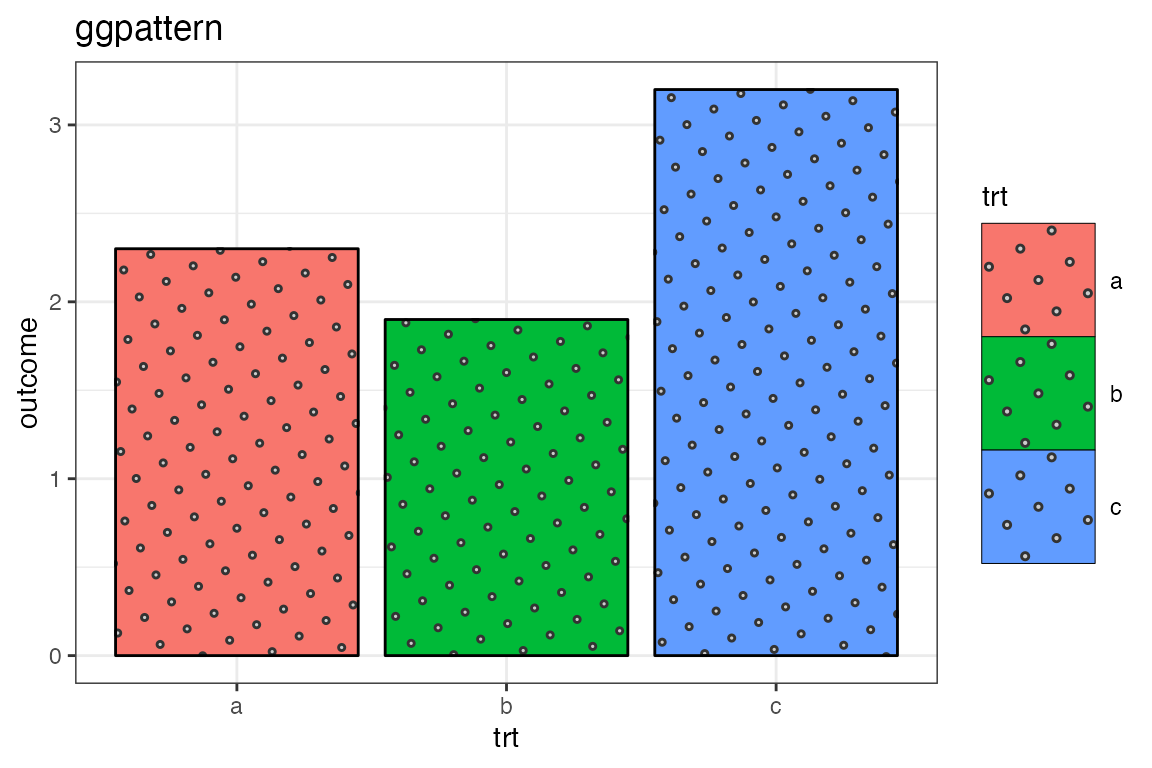## The Density Aesthetic (‘pch’ example)

The aesthetic pattern_density roughly corresponds to the fraction of the filled area which should be covered by the pattern.

In the following plot the density of striping is increased to 50% of the fill area.

ggplot(df, aes(trt, outcome)) +
geom_col_pattern(
aes(fill=trt, pattern_shape=trt),
colour          = 'black',
pattern         = 'pch',
pattern_density = 0.5
) +
theme_bw() +
labs(title = "Fixed density of 0.5 (50% of the fill area)") +
theme(legend.key.size = unit(1.5, 'cm'))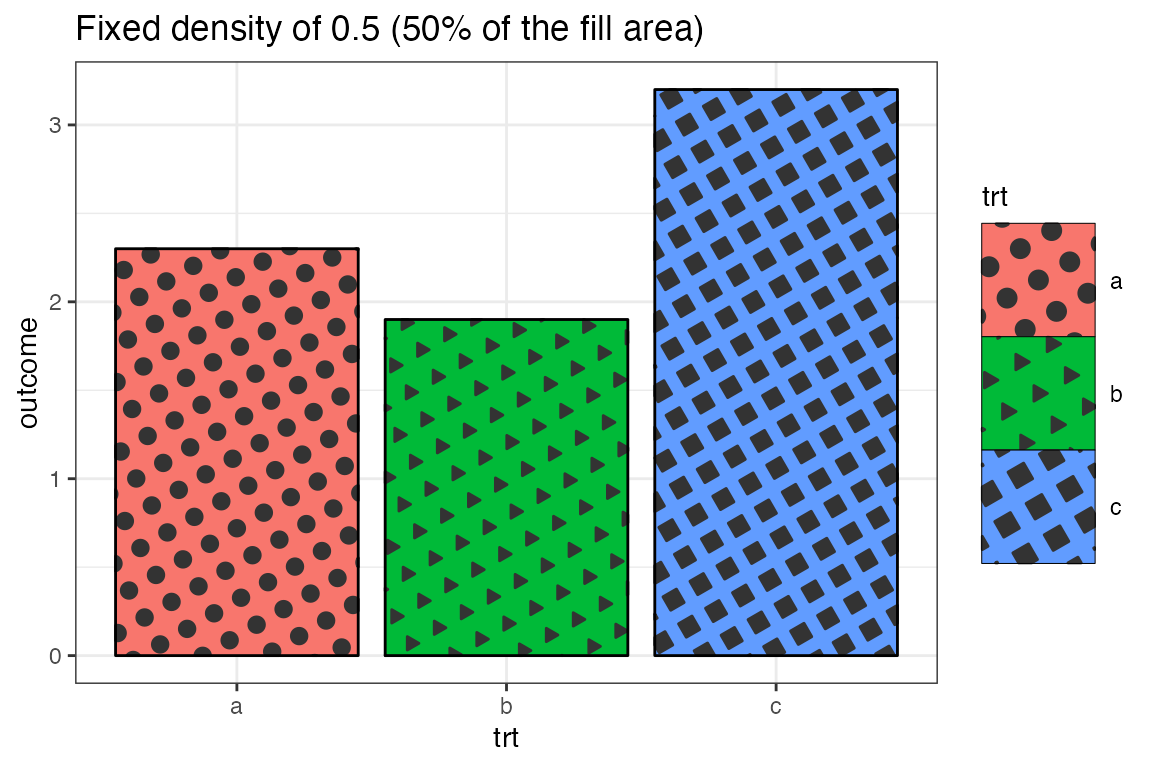## The Density Aesthetic as a Mapped Aesthetic

ggplot(df, aes(trt, outcome)) +
geom_col_pattern(
aes(fill = trt, pattern_density = trt),
colour          = 'black',
pattern         = 'circle'
) +
theme_bw() +
labs(title = "Aesthetic Mapping of 'trt' to Density") +
theme(legend.key.size = unit(1.5, 'cm'))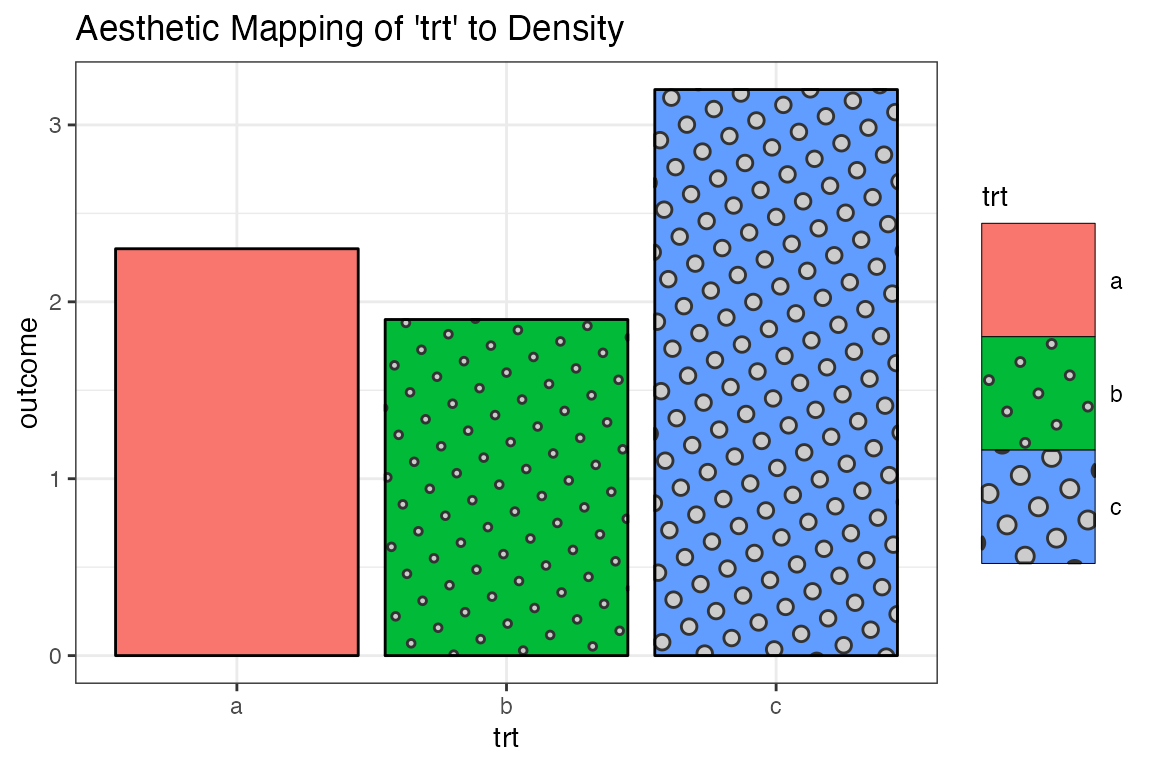## The Density Aesthetic as a Mapped Aesthetic with Manual Scale

scale_pattern_density_manual() can be used to manually control how the variable is mapped to the density.

ggplot(df, aes(trt, outcome)) +
geom_col_pattern(
aes(fill = trt, pattern_density = trt),
colour          = 'black',
pattern         = 'regular_polygon',
pattern_shape   = 'convex6',
pattern_fill    = 'white',
pattern_colour  = NA,
pattern_grid    = 'hex'
) +
theme_bw() +
labs(title = "Aesthetic Mapping of 'trt' to Density") +
theme(legend.key.size = unit(1.5, 'cm')) +
scale_pattern_density_manual(values = c(a = 0.7, b=0.8, c=0.9))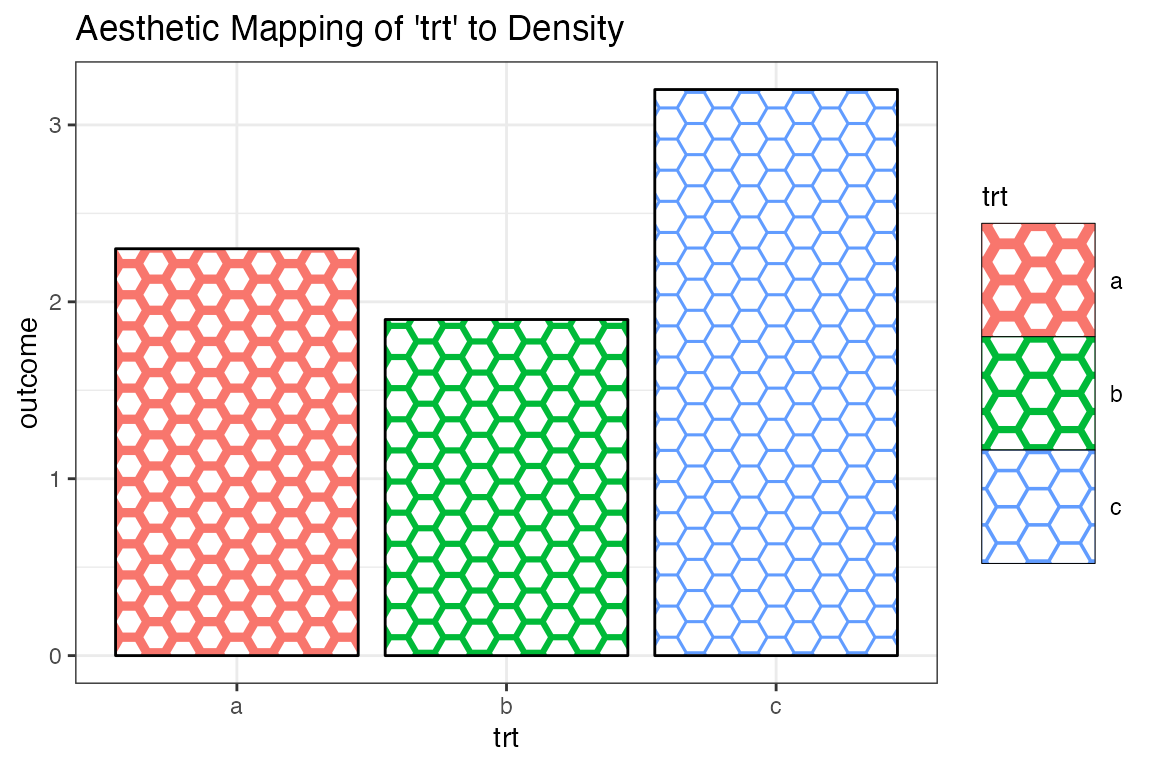## The Spacing Aesthetic as a Mapped Aesthetic

ggplot(df, aes(trt, outcome)) +
geom_col_pattern(
aes(fill = trt, pattern_spacing = trt, pattern_shape = trt),
pattern_density = 0.7,
colour          = 'black',
pattern         = 'regular_polygon',
pattern_scale   = 0.2
) +
theme_bw() +
labs(title = "Aesthetic Mapping of 'trt' to Spacing") +
scale_pattern_shape_manual(values = c(a = "circle", b = "convex3", c = "star5")) +
theme(legend.key.size = unit(1.5, 'cm'))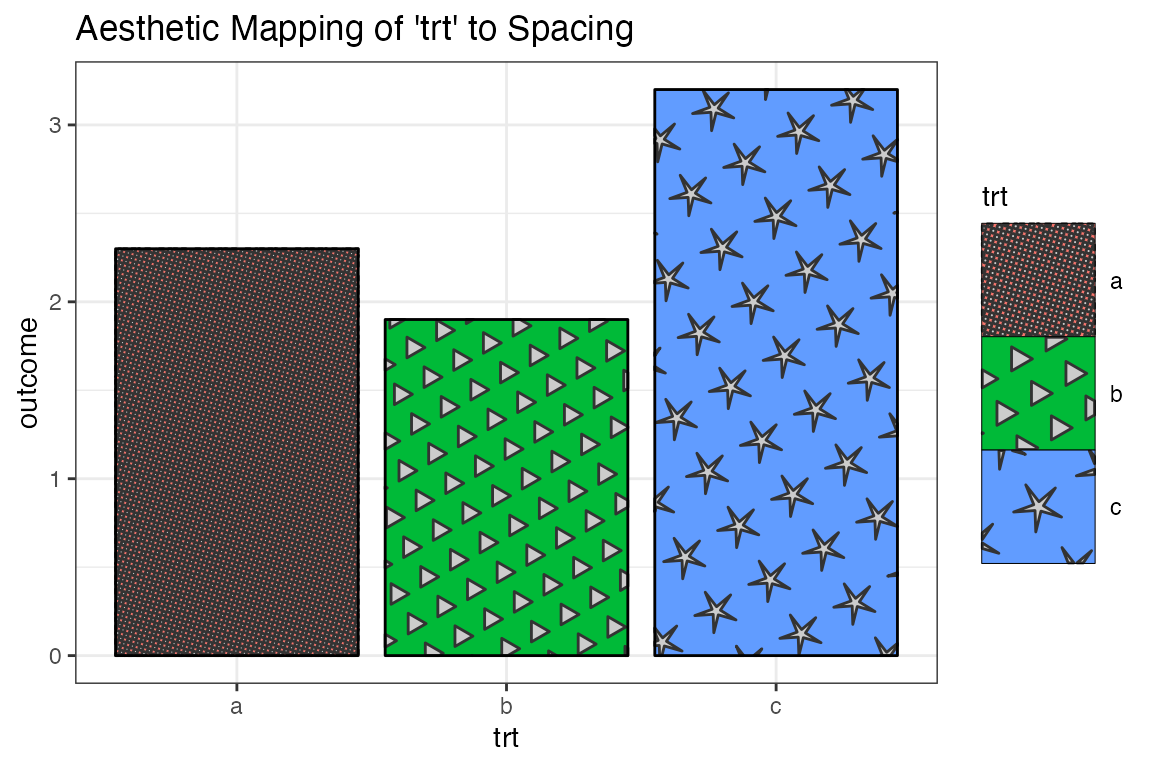## The Fill Aesthetic as a Mapped Aesthetic (‘rose’ example)

ggplot(df, aes(trt, outcome)) +
geom_col_pattern(
aes(fill = trt, pattern_fill = trt),
colour          = 'black',
pattern         = 'rose',
pattern_frequency = 5/2,
pattern_density = 0.7,
pattern_spacing = 0.15
) +
theme_bw() +
labs(title = "Aesthetic Mapping of 'trt' to Pattern Fill") +
scale_pattern_fill_viridis_d() +
theme(legend.key.size = unit(1.5, 'cm'))